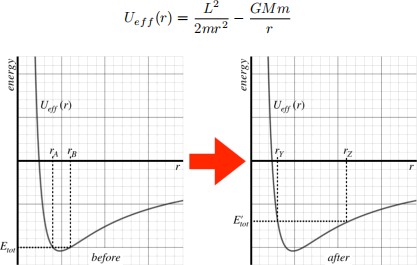Hello, dear friend, you can consult us at any time if you have any questions, add WeChat: daixieitPHY 9HA FQ2021                                                                HW #9

Fewer questions (Thanksgiving break) and due date on Wednesday!!!  We will cover ”Small oscillations” on Monday so you can wait

Q91 (multiple choice)

Consider the harmonic motion described by the graph of position vs. time. At which point is

the amplitude of the motion the greatest?

a. A

b. B

c. C

e. The amplitude is the same at all three points.

Q92

In the far future, a space station orbits the sun in an orbit where its fastest speed is nine times its slowest speed. As the space station nears the aphelion of its orbit, the “solar forecast” (remember, this is the far future) predicts solar flares that will endanger the station if it gets any closer to the sun than its aphelion. The station doesn’t have fuel to speed up its motion to keep itself from getting closer to the sun, but it has a system of hydraulics that it can use to launch any number of modular pieces of itself.  The trouble is, these modular pieces contain people & equipment that can’t simply be sacrificed to save the main station.  So the plan is to launch them backward fast enough so that both the remaining station and the ejected parts enter circular orbits in opposite directions. This way, they both stay safely far from the sun, and will meet again later (as they remain the same distance from the sun and are going in opposite directions), at which point they can re-dock the ejected modules after the solar flare danger has passed.

Q93

The orbit of a spaceship around a planet is depicted in the left-hand diagram below. It then receives a “kick” from its engines at the aphelion of the orbit changing its total orbital energy from Etot to Eot, as shown in the right diagram below. The gridlines in the two diagrams are on the same scales for both axes. The effective potential is given by the usual equation given below.  [M is the mass of the planet, m is the mass of the spaceship, and L is the angular momentum of the spaceship measured around the reference point at the center of the planet.]a. Describe as much as you can about the direction of the “kick” given to the spaceship. Explain how you know.

c. If it took the spaceship 3 hours to get from rA to rB  in its original orbit, find the time it takes it to get from rY  to rZ  after its orbit is changed.

Q94

A block vibrates on a spring on a horizontal frictionless table.  The distance between the two extreme ends of the block’s motion is 1.2 m, and it takes the block 0.80 s to traverse that distance.

b. Let the motion of the block be along the x-axis, with the equilibrium point being at the origin. Determine the equation of motion for x(t) if the clock measuring t starts when the block is at x = −0.3 m, and is moving toward the origin.